International
Tables for
Crystallography
Volume B
Reciprocal space
Edited by U. Shmueli

International Tables for Crystallography (2010). Vol. B, ch. 1.1, pp. 7-8   | 1 | 2 |

Section 1.1.5. Transformations

U. Shmuelia*

aSchool of Chemistry, Tel Aviv University, 69 978 Tel Aviv, Israel
Correspondence e-mail: ushmueli@post.tau.ac.il

1.1.5. Transformations

| top | pdf |

1.1.5.1. Transformations of coordinates

| top | pdf |

It happens rather frequently that a vector referred to a given basis has to be re-expressed in terms of another basis, and it is then required to find the relationship between the components (coordinates) of the vector in the two bases. Such situations have already been indicated in the previous section. The purpose of the present section is to give a general method of finding such relationships (transformations), and discuss some simplifications brought about by the use of mutually reciprocal and Cartesian bases. We do not assume anything about the bases, in the general treatment, and hence the tensor formulation of Section 1.1.4is not appropriate at this stage.

Letandbe the given and required representations of the vector r, respectively. Upon the formation of scalar products of equations (1.1.5.1)and (1.1.5.2)with the vectors of the second basis, and employing again the summation convention, we obtainorwhereand. Similarly, if we choose the basis vectors, l = 1, 2, 3, as the multipliers of (1.1.5.1)and (1.1.5.2), we obtainwhereand. Rewriting (1.1.5.4)and (1.1.5.5)in symbolic matrix notation, we haveleading toandandleading toandEquations (1.1.5.7)and (1.1.5.9)are symbolic general expressions for the transformation of the coordinates of r from one representation to the other.

In the general case, therefore, we require the matrices of scalar products of the basis vectors, G(12) and G(22) or G(11) and G(21) – depending on whether the basisor, k = 1, 2, 3, was chosen to multiply scalarly equations (1.1.5.1)and (1.1.5.2). Note, however, the following simplifications.

 (i) If the basesandare mutually reciprocal, each of the matrices of mixed scalar products, G(12) and G(21), reduces to a unit matrix. In this important special case, the transformation is effected by the matrices of the metric tensors of the bases in question. This can be readily seen from equations (1.1.5.7)and (1.1.5.9), which then reduce to the relationships between the covariant and contravariant components of the same vector [see equations (1.1.4.11)and (1.1.4.12)above]. (ii) If one of the bases, say, is Cartesian, its metric tensor is by definition a unit tensor, and the transformations in (1.1.5.7)reduce toandThe transformation matrix is now the mixed matrix of the scalar products, whether or not the basis, k = 1, 2, 3, is also Cartesian. If, however, both bases are Cartesian, the transformation can also be interpreted as a rigid rotation of the coordinate axes (see Chapter 3.3).

It should be noted that the above transformations do not involve any shift of the origin. Transformations involving such shifts, notably the symmetry transformations of the space group, are treated rather extensively in Volume A of International Tables for Crystallography (2005) [see e.g. Part 5there (Arnold, 2005)].

1.1.5.2. Example

| top | pdf |

This example deals with the construction of a Cartesian system in a crystal with given basis vectors of its direct lattice. We shall also require that the Cartesian system bear a clear relationship to at least one direction in each of the direct and reciprocal lattices of the crystal; this may be useful in interpreting a physical property which has been measured along a given lattice vector or which is associated with a given lattice plane. For a better consistency of notation, the Cartesian components will be denoted as contravariant.

The appropriate version of equations (1.1.5.1)and (1.1.5.2)is nowandwhere the Cartesian basis vectors are: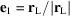,and, and the vectors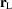andare given by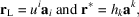whereand, i, k = 1, 2, 3, are arbitrary integers. The vectorsandmust of course be chosen to be mutually perpendicular,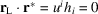. Theaxis of the Cartesian system thus coincides with a direct-lattice vector, and theaxis is parallel to a vector in the reciprocal lattice.

Since the basis in (1.1.5.12)is a Cartesian one, the required transformations are given by equations (1.1.5.10)aswhere, k, i = 1, 2, 3, form the matrix of the scalar products. If we make use of the relationships between covariant and contravariant basis vectors, and the tensor formulation of the vector product, given in Section 1.1.4above (see also Chapter 3.1), we obtain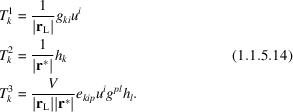Note that the other convenient choice,and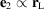, interchanges the first two columns of the matrix T in (1.1.5.14)and leads to a change of the signs of the elements in the third column. This can be done by writinginstead of, while leaving the rest ofunchanged.

References

International Tables for Crystallography (2005). Vol. A, Space-Group Symmetry, edited by Th. Hahn. Heidelberg: Springer.
Arnold, H. (2005). Transformations in crystallography. In International Tables for Crystallography, Vol. A, Space-Group Symmetry, edited by Th. Hahn, Part 5. Heidelberg: Springer.